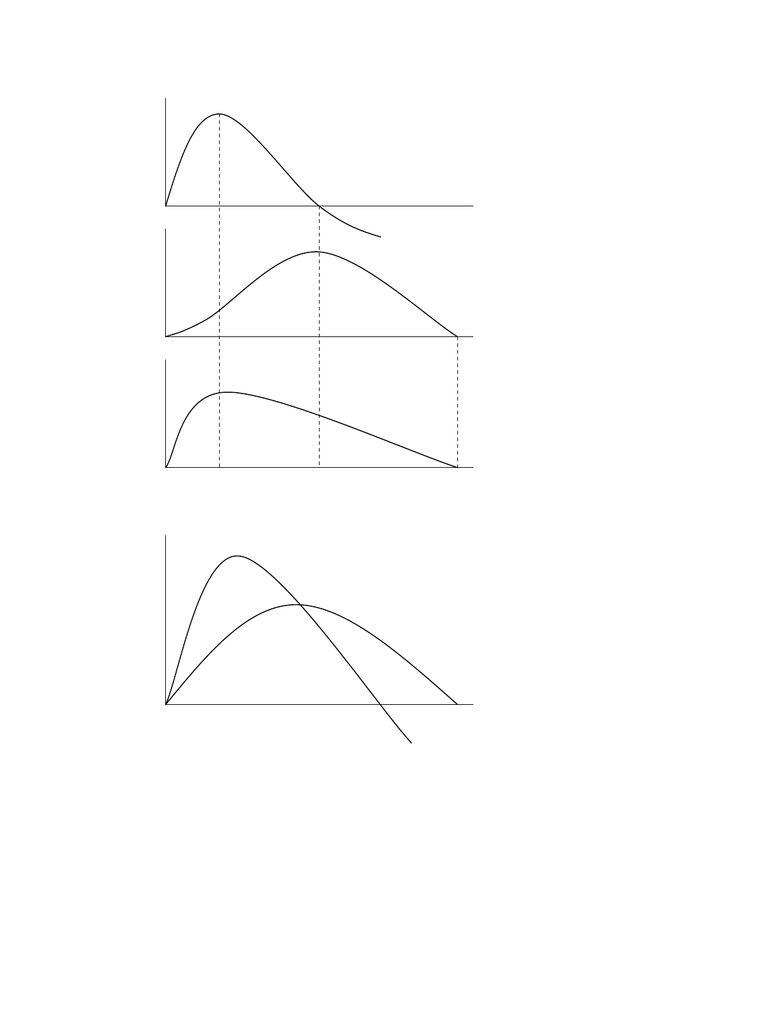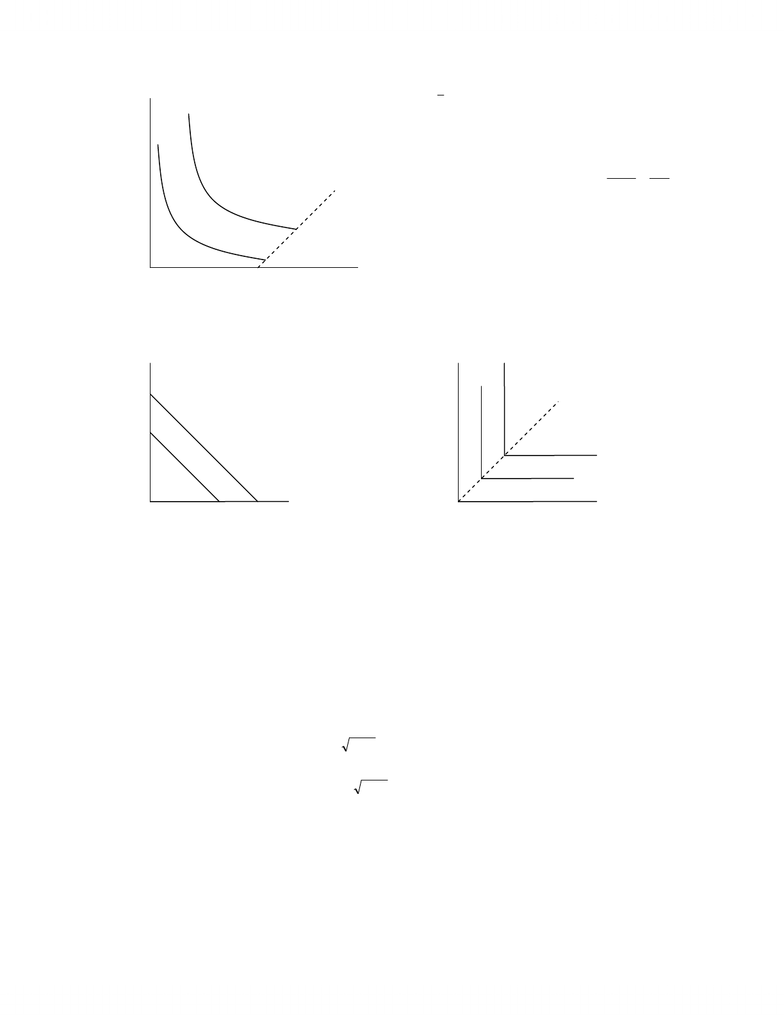# Summary notes 2End

226 views22 pages
School
Department
Course
ProfessorECO200Y1b - Theory of the Firm.doc
Page 1 of 22
Productivity Curves
Definitions
Very short run:
(
)
LKqq ,= perfectly inelastic supply curve.
Short run:
(
)
LKqq ,=.
Long run:
(
)
LKqq ,=.
Very long run:
(
)
LKqq ,= the production function q itself changes.
SHORT RUN PRODUCTIVITY CURVES
Total productivity:
(
)
LKqqTP
L
,== .
Average productivity:
L
q
L
TP
AP L
L== .
Marginal productivity:
L
q
L
TP
MPL
L
=
= decreases (diminishing marginal return).
Inputs Firm Output
Production Function
(
)
,, 21 xxqq =
www.notesolution.com
Unlock document

This preview shows pages 1-3 of the document.
Unlock all 22 pages and 3 million more documents.ECO200Y1b - Theory of the Firm.doc
Page 2 of 22
LONG RUN PRODUCTIVITY CURVES
In the long run,
(
)
LKqq ,= there are various levels of K and L to produce a specific level of output.
Isoquants
Hold q constant and see what combinations of K and L are required.
MPL
L
APL MPL
APL
APL
TPL
MPL
L
L
L
www.notesolution.com
Unlock document

This preview shows pages 1-3 of the document.
Unlock all 22 pages and 3 million more documents.ECO200Y1b - Theory of the Firm.doc
Page 3 of 22
(
)
LKqq ,=.
12 qq > q2 represents a higher output.
Negatively sloped if K decreases, L must
increase to keep q constant.
L
K
MP
MP
MRTS
K
L
KL
=== /
slope.
Convex because of the law of diminishing returns
MRTS decreases as L increases and K
decreases.
Non-Typical Isoquants
Perfect Substitutes
MRTS constant.
Fixed Proportions
MRTS = 0.
RETURNS TO SCALE
Let
(
)
LKqq ,
1= and
(
)
LKqq 2,2
1= amount of inputs are doubled.
Math Examples of Long Run Production Functions
(
)
LKLKqq == ,.
(
)
LKLKqq == ,.
(
)
3
,LKLKqq == .
Problem
Its impossible for a production function which exhibits increasing returns to scale to also adhere to the law
of diminishing returns. Do you agree?
No, it is possible. The law of diminishing returns is in the short-run, but returns to scale is in the
long-run.
L
K
q2
q1
L
K
q2
q1
ridge line
L
K
q2
q1
www.notesolution.com
Unlock document

This preview shows pages 1-3 of the document.
Unlock all 22 pages and 3 million more documents.

## Document Summary

Definitions: very short run, short run: q, long run: q, very long run: There are various levels of k and l to produce a specific level of output. Isoquants: hold q constant and see what combinations of k and l are required. Q2 represents a higher output: negatively sloped if k decreases, l must increase to keep q constant. slope. L: convex because of the law of diminishing returns. Mrts decreases as l increases and k decreases. ridge line q2 q1. L: mrts constant, mrts = 0. q2 q1. 1 = q and: constant return to scale: (output doubled). Increasing return to scale: decreasing return to scale: (output more than doubled). (output less than doubled). Math examples of long run production functions: constant return to scale: q. It"s impossible for a production function which exhibits increasing returns to scale to also adhere to the law of diminishing returns" . Do you agree: no, it is possible.

## Get access